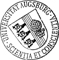## Model reduction of descriptor systems by interpolatory projection methods

• In this paper, we investigate interpolatory projection framework for model reduction of descriptor systems. With a simple numerical example, we first illustrate that employing subspace conditions from the standard state space settings to descriptor systems generically leads to unbounded H2 error due to the mismatch of the polynomial parts of the full and reduced-order transfer functions. We then develop modified interpolatory subspace conditions based on the deflating subspaces that guarantee a bounded error. For the special cases of index-1 and index-2 descriptor systems, we also show how to avoid computing these deflating subspaces explicitly while still enforcing interpolation. The question of how to choose interpolation points ptimally naturally arises as in the standard state space setting. We answer this question in the framework of the H2-norm by extending the Iterative Rational Krylov Algorithm (IRKA) to descriptor systems. Several numerical examples are used to illustrate theIn this paper, we investigate interpolatory projection framework for model reduction of descriptor systems. With a simple numerical example, we first illustrate that employing subspace conditions from the standard state space settings to descriptor systems generically leads to unbounded H2 error due to the mismatch of the polynomial parts of the full and reduced-order transfer functions. We then develop modified interpolatory subspace conditions based on the deflating subspaces that guarantee a bounded error. For the special cases of index-1 and index-2 descriptor systems, we also show how to avoid computing these deflating subspaces explicitly while still enforcing interpolation. The question of how to choose interpolation points ptimally naturally arises as in the standard state space setting. We answer this question in the framework of the H2-norm by extending the Iterative Rational Krylov Algorithm (IRKA) to descriptor systems. Several numerical examples are used to illustrate the theoretical discussion.Author: Serkan Gugercin, Tatjana StykelGND, Sarah Wyatt urn:nbn:de:bvb:384-opus4-22155 https://opus.bibliothek.uni-augsburg.de/opus4/2215 Preprints des Instituts für Mathematik der Universität Augsburg (2013-03) Preprint English Universität Augsburg 2013/01/29 interpolatory model reduction; H2 optimal approximation; descriptor systems; differential algebraic equations Differential-algebraisches Gleichungssystem; Ordnungsreduktion; Optimale Interpolation; Numerisches Verfahren `Erschienen in: SIAM J. Sci. Comput., 35(5), B1010–B1033, https://doi.org/10.1137/130906635` Mathematisch-Naturwissenschaftlich-Technische Fakultät Mathematisch-Naturwissenschaftlich-Technische Fakultät / Institut für Mathematik Mathematisch-Naturwissenschaftlich-Technische Fakultät / Institut für Mathematik / Lehrstuhl für Numerische Mathematik 5 Naturwissenschaften und Mathematik / 51 Mathematik / 510 MathematikDeutsches Urheberrecht